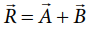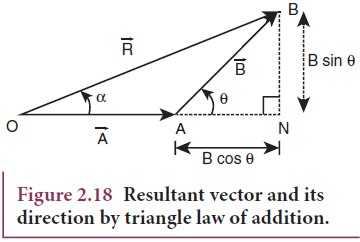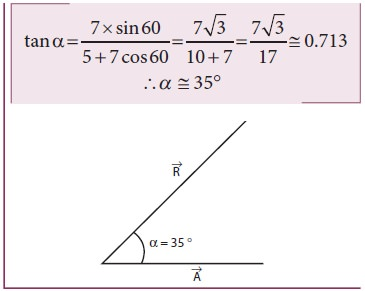Home | | Physics 11th std | Addition of Vectors (Triangular Law of addition method)

## Chapter: 11th Physics : UNIT 2 : Kinematics

Since vectors have both magnitude and direction they cannot be added by the method of ordinary algebra.

Since vectors have both magnitude and direction they cannot be added by the method of ordinary algebra. Thus, vectors can be added geometrically or analytically using certain rules called ‘vector algebra’. In order to find the sum (resultant) of two vectors, which are inclined to each other, we use (i) Triangular law of addition method or (ii) Parallelogram law of vectors.

## Triangular Law of a͢ddition method

Let us consider two vectorsandas shown in Figure 2.16.To find the resultant of the two vectors we apply the triangular law of addition as follows:

Represent the vectorsandby the two adjacent sides of a triangle taken in the same order. Then the resultant is given by the third side of the triangle as shown in Figure 2.17.To explain further, the head of the first vectoris connected to the tail of the second vector. Let θ be the angle betweenand. Thenis the resultant vector connecting the tail of the first vectorto the head of the second vector. The magnitude of(resultant) is given geometrically by the length of(OQ) and the direction of the resultant vector is the angle betweenand. Thus we write.1. Magnitude of resultant vector

The magnitude and angle of the resultant vector are determined as follows.

From Figure 2.18, consider the triangle ABN, which is obtained by extending the side OA to ON. ABN is a right angled triangle.From Figure 2.18For OBN, we have OB2 = ON 2 + BN 2which is the magnitude of the resultant ofand2. Direction of resultant vectors:

If θ is the angle betweenand, then## Solved Example Problem for Addition of VectorsTags : with Solved Example Problems , 11th Physics : UNIT 2 : Kinematics
Study Material, Lecturing Notes, Assignment, Reference, Wiki description explanation, brief detail
11th Physics : UNIT 2 : Kinematics : Addition of Vectors (Triangular Law of addition method) | with Solved Example Problems